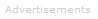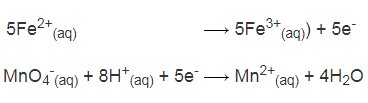# Definition of Redox Reactions (balancing)

To Balance a Redox Reaction Equation
Carry out the following steps. This can seem a little complicated at first, but it helps to look at the example lower on the page:
1. Write out the unbalanced oxidation and reduction half equations.
2. Balance each half equation.
3. Balance the elements being oxidized and reduced, ignoring H and O at this stage.
4. Balance O atoms by adding H2O.
5. Then balance H by either:
• adding H+ (for acidic solutions) or
• adding OH- (for basic solutions).
6. Balance charge on the left vs the right side of each half equation by adding electrons if needed.
7. Multiply the half equations by appropriate factors so that each involves the transfer of the same number of electrons.
8. Combine the half reactions to eliminate the electrons from the overall reaction.### Example

Here is a redox reaction in acid solution that we're going to balance:

Fe2+(aq) + MnO4-(aq) → Fe3+(aq) + Mn2+(aq)

Step A
Write the unbalanced half equations:

Fe2+(aq) → Fe3+(aq) (oxidation)

MnO4-(aq) → Mn2+(aq) (reduction)

Step B
Balance each half reaction.

Iron Half Reaction: To balance this equation, we simply need to add an electron to the right side of the equation.

Fe2+(aq) → Fe3+(aq)) + e-

Permanganate Half Reaction: We add water to the right to balance the four oxygens on the left.

MnO4-(aq) → Mn2+(aq) + 4H2O

Next, to balance the eight hydrogens in the water, we add 8H+(aq) on the left.

MnO4-(aq) + 8H+(aq) → Mn2+(aq) + 4H2O

Now, looking at the equation above, we can see the charges are unbalanced on the left vs the right side. The overall charge on the left is +7 and on the right is +2. To balance the charge, we need to add five electrons to the left side of the equation.

MnO4-(aq) + 8H+(aq) + 5e- → Mn2+(aq) + 4H2O

Step C
Multiply the half equations by appropriate numbers so they each contain the same number of free electrons on each side. In this case, we must multiply the iron reaction by 5.Step D
Add the half reactions. The electrons on each side cancel to give the overall reaction:

5Fe2+(aq) + MnO4-(aq) + 8H+(aq) → 5Fe3+(aq)) + Mn2+(aq) + 4H2OSearch the Dictionary for More Terms